# How to Create Dynamic Drop Down List without Blank in Excel

This post will guide you how to create dynamic drop down list without blank cells in Microsoft Excel.

In Excel, and you can use Data Validation feature to improve the efficiency of data entry in excel, and it also be used to reduce mistake and typing errors. And it is also be used to restrict the user for the type of data that can be entered in the range.

Assuming that you have a list of data in your current worksheet, and you can want to create a dynamic drop down list based on those data without blank cells, and if you use the usual method to create it, and it may be doesn’t work. And you can use an OFFSET formula and count the entries in the column, and calculate the number of rows in the range, and then create a source list without blanks, and use formulas to pull the numbered items into a new column. Just do the following steps:

Step1: select cell A2 next to the original data list B1:B8, and type the following formula in Cell A2, and copy it down to cell A8, and it will number the cells that are not blank.

=IF(B2=””,””,MAX(A\$1:A1)+1)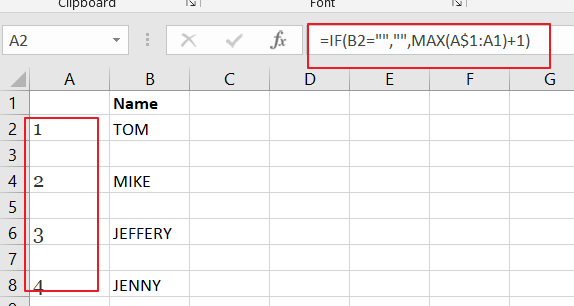Step2: then you can create a new source list column without blanks based on the source data list, just enter the following formula into the cell D2 in a new column, and then copy it down to the cell D8 to create a new data list with all the blanks at the end.

=IFERROR(INDEX(\$B\$2:\$B\$8,MATCH(ROW()-ROW(\$D\$1),\$A\$2:\$A\$8,0)),””)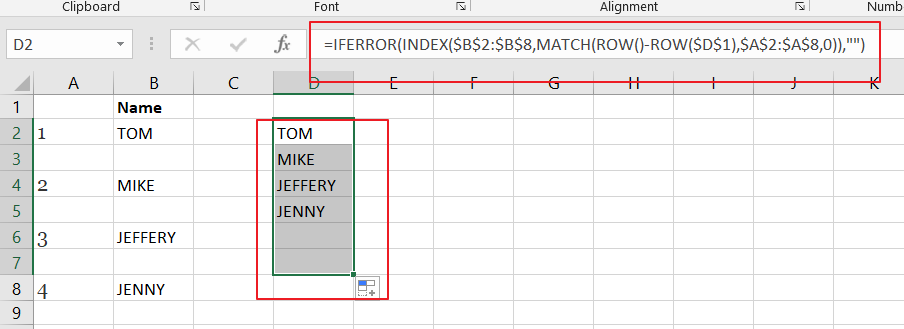Step3: you can create a dynamic drop down list based on the newly source range without blanks. Select a cell that you want to create dynamic drop down list , and go to Data tab, and click Data Validation command under Data Tools group. And the Data Validation dialog will appear.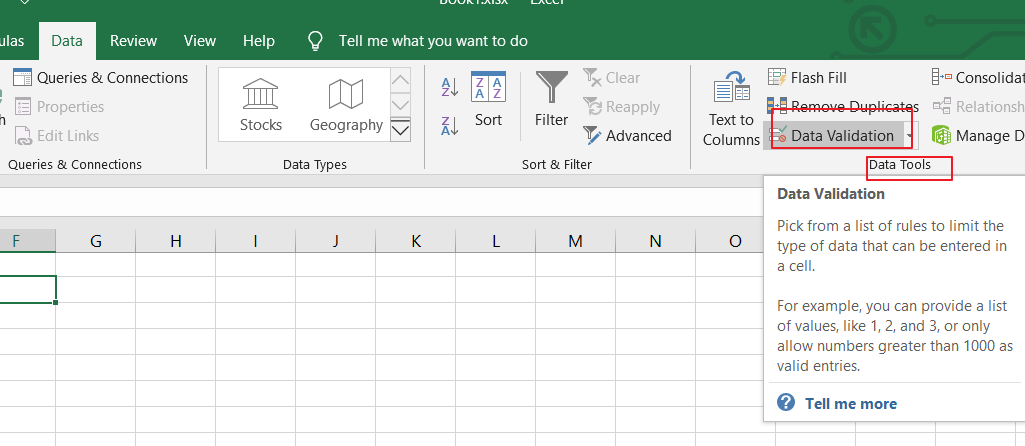Step4: select List value from the Allow drop down list in the Data Validation dialog box, and enter the following formula into the Source text box. And click Ok button.

=OFFSET(\$D\$1,1,0,MAX(\$A:\$A),1)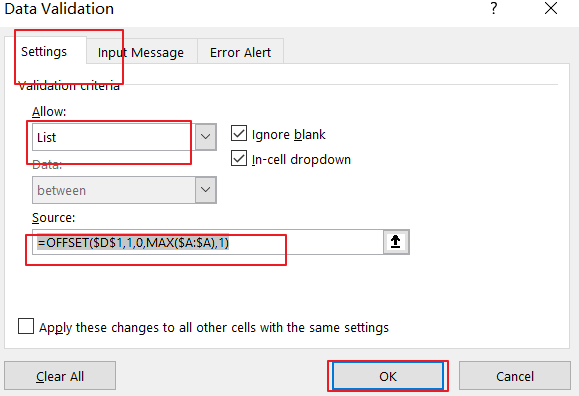Step5: You would see that the dynamic drop down list should be created without blanks in your current worksheet.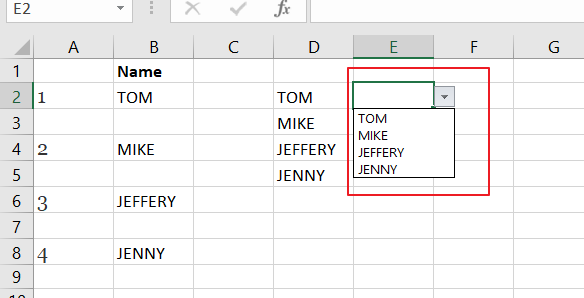### Related Functions

• Excel IF function
The Excel IF function perform a logical test to return one value if the condition is TRUE and return another value if the condition is FALSE. The IF function is a build-in function in Microsoft Excel and it is categorized as a Logical Function.The syntax of the IF function is as below:= IF (condition, [true_value], [false_value])….
• Excel IFERROR function
The Excel IFERROR function returns an alternate value you specify if a formula results in an error, or returns the result of the formula.The syntax of the IFERROR function is as below:= IFERROR (value, value_if_error)….
• Excel INDEX function
The Excel INDEX function returns a value from a table based on the index (row number and column number)The INDEX function is a build-in function in Microsoft Excel and it is categorized as a Lookup and Reference Function.The syntax of the INDEX function is as below:= INDEX (array, row_num,[column_num])…
• Excel MATCH  function
The Excel MATCH function search a value in an array and returns the position of that item.The MATCH function is a build-in function in Microsoft Excel and it is categorized as a Lookup and Reference Function.The syntax of the MATCH function is as below:= MATCH  (lookup_value, lookup_array, [match_type])….
• Excel MAX function
The Excel MAX function returns the largest numeric value from the numbers that you provided. Or returns the largest value in the array.= MAX(num1,[num2,…numn])…
• Excel ROW function
The Excel ROW function returns the row number of a cell reference.The ROW function is a build-in function in Microsoft Excel and it is categorized as a Lookup and Reference Function.The syntax of the ROW function is as below:= ROW ([reference])….

Sidebar